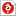검색어 입력폼

# [프로그램] 수치해석 프로그램

등록일 2002.11.26한컴오피스 (hwp) | 4페이지 | 가격 500원

없음

## 본문내용

PROGRAM D2R3
C Driver for routine LUBKSB
PARAMETER (NP=100)
DIMENSION A(NP,NP),B(NP,NP),INDX(NP)
DIMENSION C(NP,NP),X(NP)
CHARACTER TXT*3
OPEN(5,FILE='MATRX1.DAT',STATUS='OLD')
C Save matrix A for later testing
DO 12 L=1,N
DO 11 K=1,N
C(K,L)=A(K,L)
11 CONTINUE
12 CONTINUE
C Do LU decomposition
CALL LUDCMP(C,N,NP,INDX,P)
C Solve equations for each right-hand vector

없음